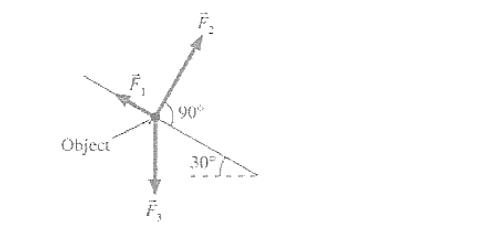Problem: Three forces are exerted on an object placed on a tilted floor, as shown in the figure. Assuming the forces have magnitude F1 = 1N, F2 = 8N, F3 = 7 N, what is the component of the net force Fnet = F1 + F2 + F3  parallel to the floor?(Take the +i axis parallel to the plane and pointing downhill.)

FREE Expert Solution
83% (177 ratings)
Problem Details

Three forces are exerted on an object placed on a tilted floor, as shown in the figure. Assuming the forces have magnitude F1 = 1N, F2 = 8N, F3 = 7 N, what is the component of the net force Fnet = F+ F+ F3  parallel to the floor?(Take the +i axis parallel to the plane and pointing downhill.)